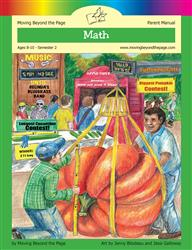Age 5-7 Reading Is Now Available!

# Common Core Alignment

CCSS.Math.Content.4.NF.3.c - Add and subtract mixed numbers with like denominators, e.g., by replacing each mixed number with an equivalent fraction, and/or by using properties of operations and the relationship between addition and subtraction.

## 5: MathUnit 5: Fractions
Lesson 10: Adding Mixed Numbers
Lesson 11: Subtracting Mixed Numbers